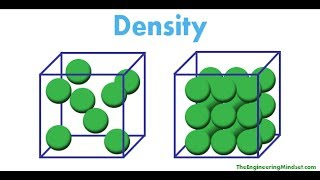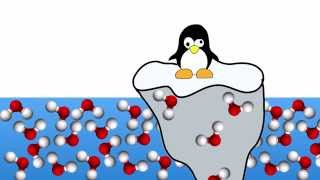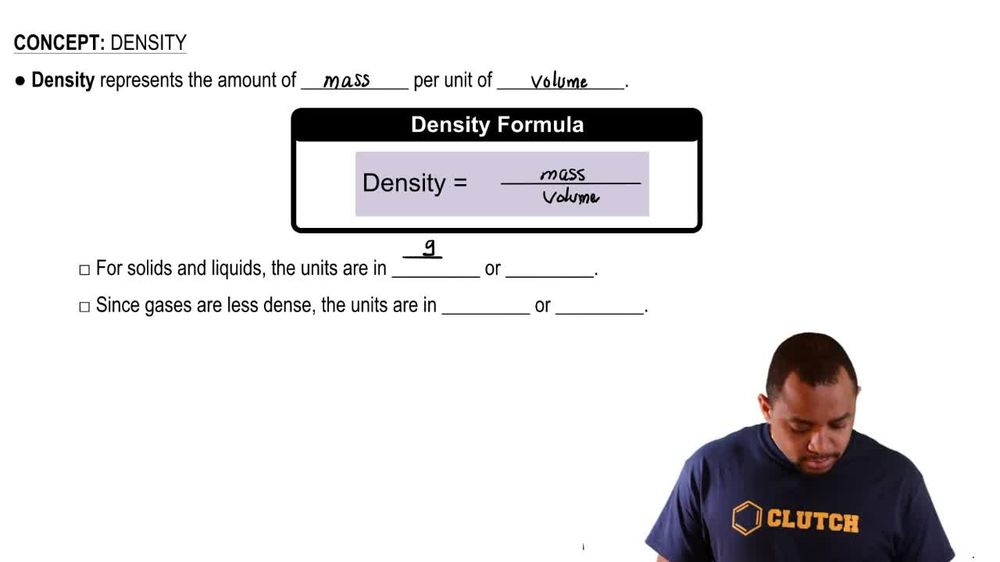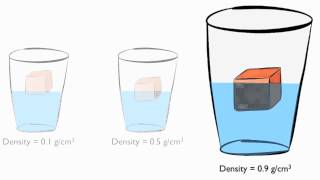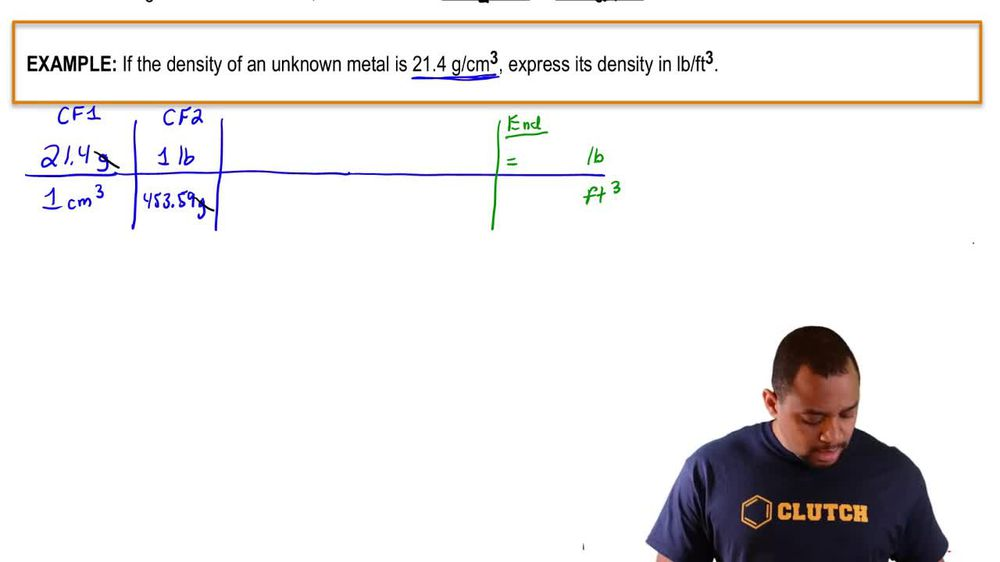Start typing, then use the up and down arrows to select an option from the list.
1. 1. Intro to General Chemistry2. Density
Problem

# Human fat has a density of 0.918 g>cm3. How much volume (in cm3) is gained by a person who gains 10.0 lb of pure fat? the reliability of a Measurement and Significant Figures

Relevant Solution1m
Play a video:
Hi everyone today, we have a question telling us that a chemical reaction for an experiment needs 53. g of painting. However, the balance in the lab was taken for maintenance and is therefore unavailable. It was decided to use a pipette to measure the painting, calculate the volume needed if the density of plantain is 0.6-6 g per milliliter. So we're going to use the formula density equals mass over volume, and we want to solve for volume. So volume is going to equal mass, divided by density and now plugging in what we know. Our volume Is going to equal 53 0.4 g, divided by zero . ml Which equals .3 Mil leaders. And that is our final answer. Thank you for watching. Bye.